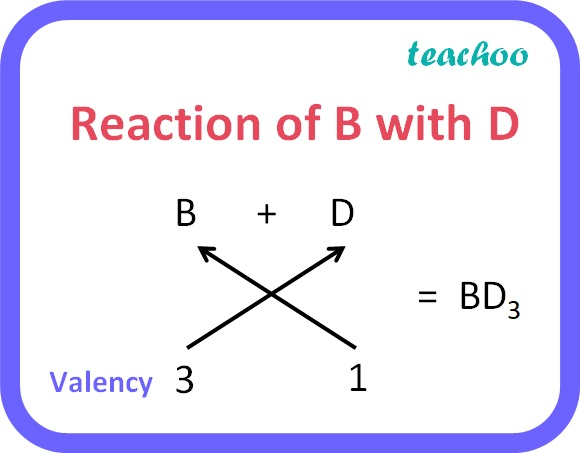Past Year - 5 Mark Questions

Class 10
Chapter 5 Class 10 - Periodic Classification of Elements

## The electrons in the atoms of four elements A, B, C and D are distributed in three shells having 1, 3, 5 and 7 electrons respectively in their outermost shells. Write the group numbers in which these elements are placed in the Modern Periodic Table. configuration of the atoms of B and D and the molecular formula of the compound formed when B and D combine.

Group number =

• 10 + number of valence electrons for p block elements
• group number = number of valence electrons for s block elements.
 A B C D Number of Valence electrons 1 3 5 7 Group Number 1 (10+3)=13 (10+5)=15 (10+7)=17

The electronic configuration of:

• B will be 2, 8, 3
• D will be 2, 8, 7

Compound formed when B and D combine:

B has a valency of 3 whereas C has a valency of 1.So, the compound formed by B and D will be BD 3

Learn in your speed, with individual attention - Teachoo Maths 1-on-1 Class